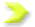RPM & LINE-SPEED METER

 Please use the free Acrobat Reader®   software, You can view and print   Adobe PDF files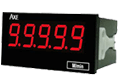Microcomputer Speed & Line Speed Display Meter   Features   1. Model: MR 2. Accuracy 0.01% of full scale 3. Measurable DC pulse wave/magnetic sensor signal 4. Maximum input frequency 50KHz 5. Rotation speed and linear speed can be set arbitrarily 6. Line speed unit meters, feet, yards/minute can be set arbitrarily 7. The sensor input pulse number can be set arbitrarily 8. The position of the decimal point can be set arbitrarily 9. Small size and high stability 10. CE certificationDual Input Speed & Line Speed Calculation Meter   Features   1. Model: MMX-L 2. Accuracy 0.1% full scale 3. LED 0.8" can measure DC pulse wave/magnetic sensor signal 4. Double input calculation function (B-A, B/A, (B/A)-1, B/(A+B)) 5. Maximum input frequency 50KHz 6. Rotation speed, line speed can be set arbitrarily (1 to 99999 pulse/rotation) 7. The sensor input pulse wave can be set arbitrarily 8. Line speed in meters, feet, yards/minute can be set arbitrarily 1 set of alarm function 9. Small size and high stability 10. CE certification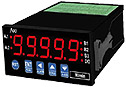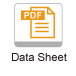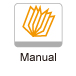Microcomputer Speed & Line Speed Display Control Meter   Features   1. Model: MMR 2. Accuracy 0.03% of full scale 3. Measurable DC pulse, magnetic sensor signal 4. Maximum input frequency 50KHz 5. Rotation speed and line speed can be set arbitrarily 6. The sensor input pulse number can be set arbitrarily 7. Two groups of alarm functions 8. Multi-level analog output switching function 9. 15 BIT analog output function 10. RS-485 interface 11. CE certification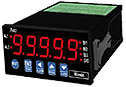Dual Input Speed & Line Speed Calculation Meter　   Features   1. Model: MMX-R 2. Accuracy 0.03% of full scale 3. It can measure DC pulse wave/magnetic sensor signal 4. Double input calculation function (B-A, B/A, (B/A)-1, B/(A+B)) 5. Maximum input frequency 50KHz 6. Rotation speed, line speed can be set arbitrarily 7. The sensor input pulse wave can be set arbitrarily 8. Line speed in meters, feet, yards/minute can be set arbitrarily     2 groups of alarm functions 9. 15bit analog output function 10. RS-485 communication interface 11. CE certification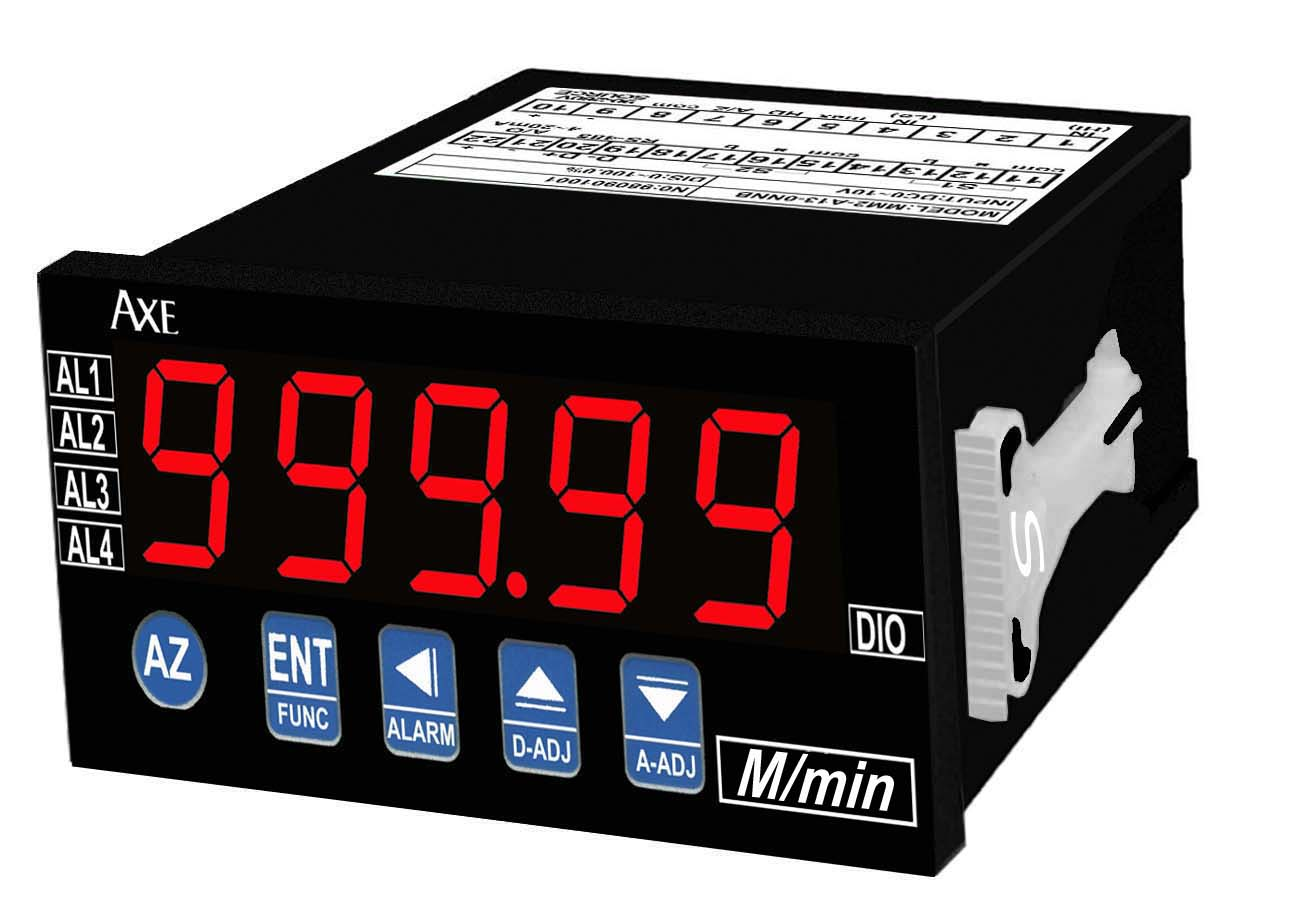Dual Input Speed & Line Speed Calculation Meter 　   Features   1. Model: MMRH 2. Accuracy 0.03% of full scale 3. LED 0.8" can measure DC pulse wave/magnetic sensor signal 4. Double input calculation function (A, B, B-A, B/A, (B/A)-1, B/(A+B)) 5. Maximum input frequency 200KHz 6. Rotation speed, line speed can be set arbitrarily 7. The sensor input pulse wave can be set arbitrarily 8. Line speed in meters, feet, yards/minute can be set arbitrarily      2 groups of alarm functions 9. 15bit analog output function 10. Two groups of alarm functions 11. RS-485 communication interface 12. CE certification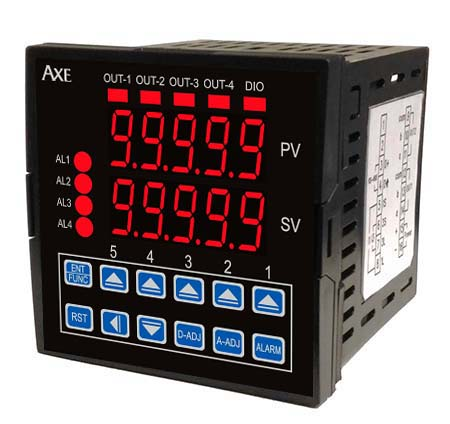Dual Input Speed & Line Speed Calculation Meter 　 (72X72mm)   Features   1. Model: MR726 2. Accuracy 0.03% of full scale 3. LED 0.8" can measure DC pulse wave/magnetic sensor signal 4. Double input calculation function (A, B, B-A, B/A, (B/A)-1, B/(A+B)) 5. Maximum input frequency 200KHz 6. Rotation speed, line speed can be set arbitrarily 7. The sensor input pulse wave can be set arbitrarily 8. Line speed in meters, feet, yards/minute can be set arbitrarily     2 groups of alarm functions 9. 15bit analog output function 10. RS-485 communication interface 11. CE certification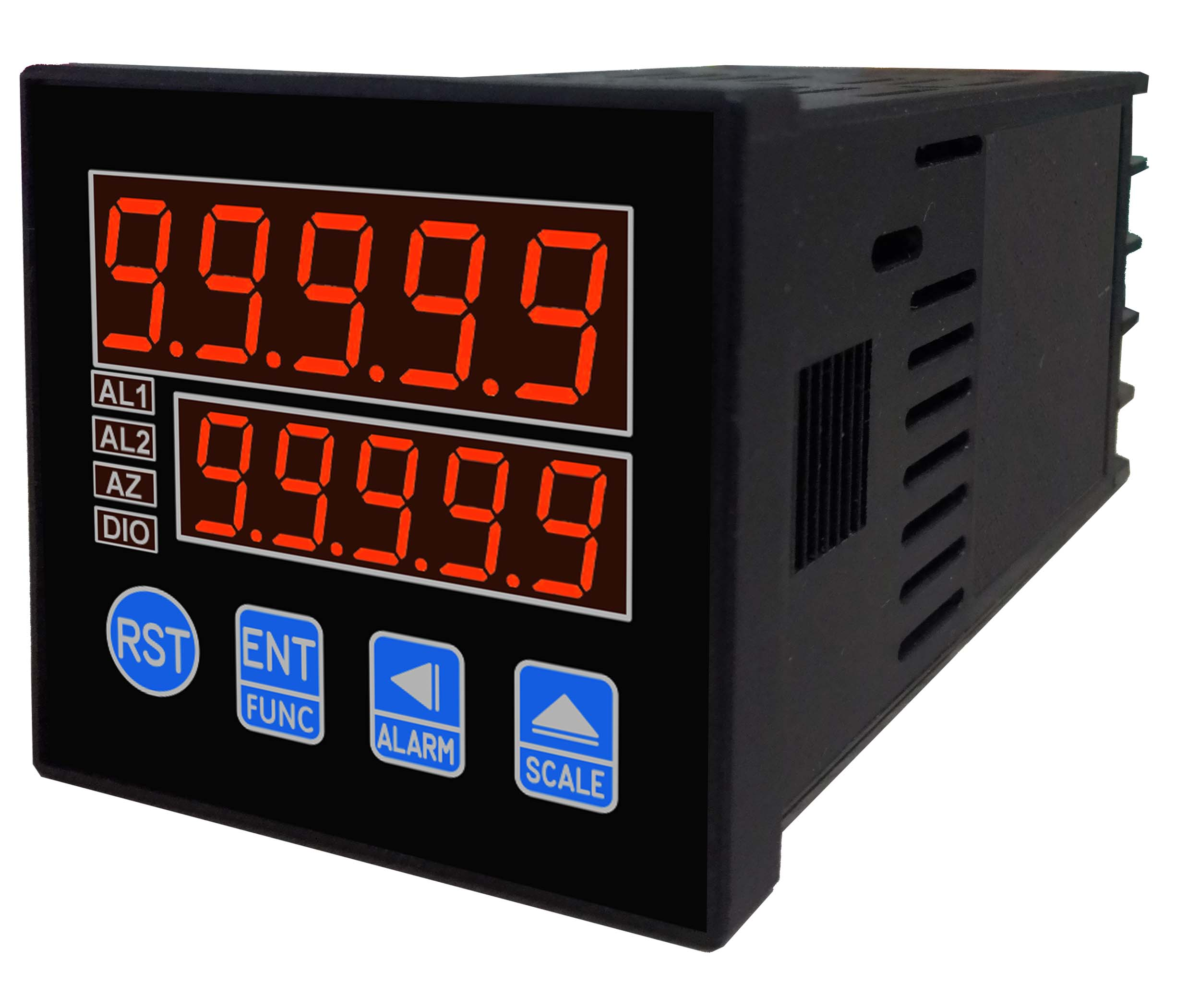Dual Input Speed & Line Speed Calculation Meter 　 (48X48mm)   Features   1. Model: MR48 2. Accuracy 0.1% full scale 3. LED 0.8" can measure DC pulse wave/magnetic sensor signal 4. Double input calculation function (B-A, B/A, (B/A)-1, B/(A+B)) 5. Maximum input frequency 50KHz 6. RPM speed, LINE line speed can be set arbitrarily     (1 to 99999 pulse/rotation) 7. The sensor input pulse wave can be set arbitrarily 8. Line speed in meters, feet, yards/minute can be set arbitrarily     2 groups of alarm functions 9. 15bit analog output function 10. RS-485 communication interface 11. CE certification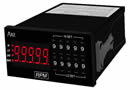Microcomputer Speed & Line Speed Control Meter (Dip Switch Setting)   Features   1. Model:MMRP 2. Accuracy 0.03% of full scale 3. It can measure DC pulse wave and magnetic sensor signal 4. Maximum input frequency 50KHz 5. Double input calculation function 6. Rotation speed, line speed can be set arbitrarily 7. The sensor input pulse number can be arbitrarily set from 1 to 99999 8. Two groups of alarm functions 9. 16BIT analog output function 10. RS-485 interface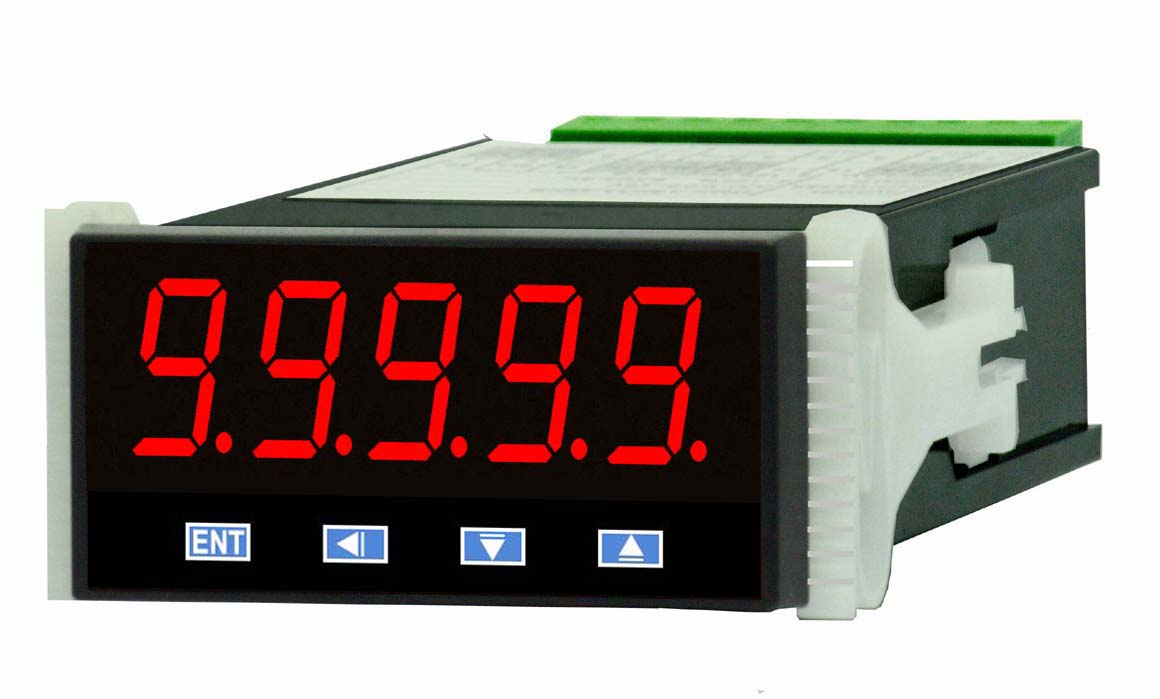Microcomputer Speed & Line Speed Control Meter   Features   1. Model: MRS-S 2. Accuracy 0.03% of full scale 3. Measurable DC pulse, magnetic sensor signal 4. Maximum input frequency 50KHz 5. Rotation speed and line speed can be set arbitrarily 6. The sensor input pulse number can be set arbitrarily 7. Two groups of alarm functions 8. 15 BIT analog output function 9. RS-485 interface 10. CE certification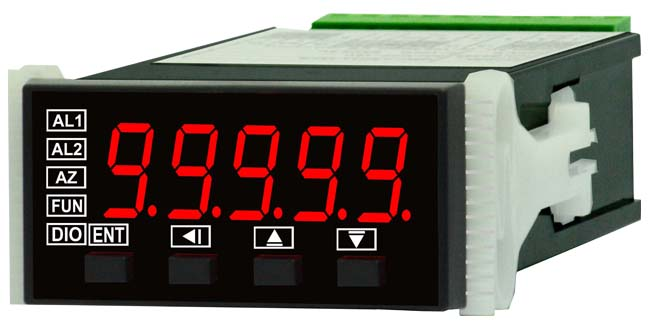Microcomputer Speed & Line Speed Meter (24X48mm)   Features   1. Model: MRS 2. Accuracy 0.01% of full scale 3. Measurable DC pulse wave/magnetic sensor signal 4. Maximum input frequency 50KHz 5. Rotation speed and linear speed can be set arbitrarily 6. Line speed unit meters, feet, yards/minute can be set arbitrarily 7. The sensor input pulse number can be set arbitrarily 8. The position of the decimal point can be set arbitrarily 9. Small size and high stability 10. CE certification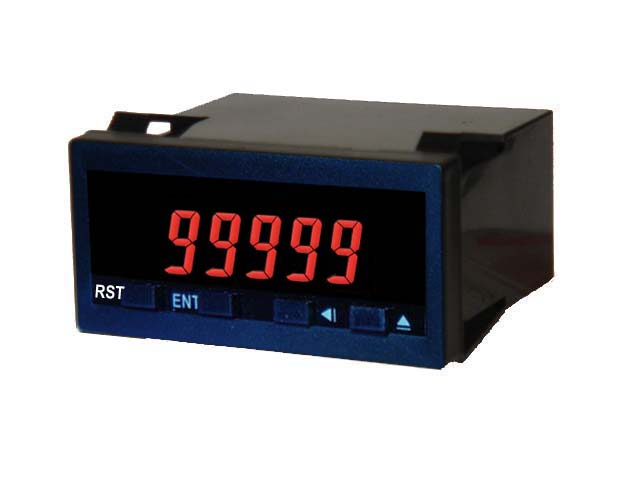Microcomputer Speed & Line Speed Meter (Low Power) (24x48mm)   Features   1. Model: MR24 2. Accuracy 0.01% of full scale 3. Maximum input frequency 50KHz 4. Rotation speed and line speed can be set arbitrarily 5. Line speed in meters, feet, yards/minute can be set arbitrarily 6. The sensor input pulse number (1-99999) can be set arbitrarily 7. Small size (24x48x48.5mm), high stability 8. Separate terminal, easy wiring 9. CE certification

 WINLING TECHNOLOGY LTD. 9F.-8,No.16,Ln.609,Sec.5,Chongxin Rd., Sanchong City,New Taipei City 24159,Taiwan  Tel:886-2-29996610   Fax:886-2-29996620  E-mail: dent.csn@msa.hinet.net    Copyright 1996-2002 WINLING Technology, Inc. All rights reserved.  Best view with 800 x 600 resolution & 16.7 million colors.